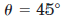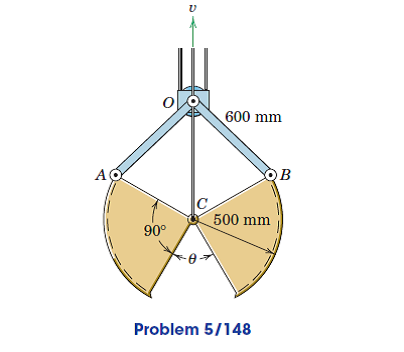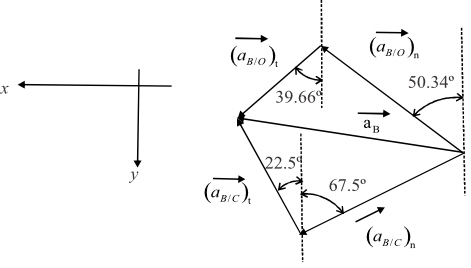Problem

# The elements of a simplified clam-shell bucket for a dredge are shown. With the block at...

The elements of a simplified clam-shell bucket for a dredge are shown. With the block at O considered fixed and with the constant velocity of the control cable at C equal to 0.5 m/s determine the angular acceleration of the right-hand bucket jaw whenas the bucket jaws are closing.#### Step-by-Step Solution

Solution 1

In $$\triangle \mathrm{OBC}$$, apply sine law and calculate the angle made by link $$O B$$.

\begin{aligned} \frac{\overline{B C}}{\sin \beta} &=\frac{\overline{O B}}{\sin 67.5^{\circ}} \\ \sin \beta &=\frac{0.5 \times \sin 67.5^{\circ}}{0.6} \\ \beta &=\sin ^{-1}\left(\frac{0.5 \times \sin 67.5^{\circ}}{0.6}\right) \\ &=50.34^{\circ} \end{aligned}

Determine the angle $$\angle O B C$$ using relation,

\begin{aligned} \angle O B C &=180^{\circ}-\left(67.5^{\circ}+50.34^{\circ}\right) \\ &=62.16^{\circ} \end{aligned}

Apply sine law and calculate the magnitude $$\overline{O C}$$.

\begin{aligned} \frac{\overline{O C}}{\sin 62.16^{\circ}} &=\frac{O B}{\sin 67.5^{\circ}} \\ \overline{O C} &=\frac{0.6 \times \sin 62.16^{\circ}}{\sin 67.5^{\circ}} \\ &=0.5742 \mathrm{~m} \end{aligned}

Calculate the magnitude for $$\overline{C C_{1}}$$ and $$\overline{O C_{1}}$$ from $$\Delta O C C_{1}$$ as follows:

\begin{aligned} \overline{C C_{1}} &=\overline{O C} \tan \beta \\ &=0.5742 \times \tan 50.34^{\circ} \\ &=0.6926 \mathrm{~m} \end{aligned}

Determine the magnitude of $$\left(\overline{O C_{1}}\right)$$.

\begin{aligned} \overline{O C_{1}} &=\frac{\overline{O C}}{\cos \beta} \\ &=\frac{0.5742}{\cos 50.34^{\circ}} \\ &=0.8996 \mathrm{~m} \end{aligned}

From geometry calculate the length of $$\overline{B C_{1}}$$ as follows:

\begin{aligned} \overline{B C_{1}} &=\overline{O C_{1}}-\overline{O B} \\ &=(0.8996-0.6) \\ &=0.2996 \mathrm{~m} \end{aligned}

Calculate the angular velocity of bucket $$\left(\omega_{B C}\right)$$ using relation,

$$\omega_{B C}=\frac{v_{e}}{\overline{C C_{1}}}$$

Substitute, $$0.5 \mathrm{~m} / \mathrm{s}$$ for $$v_{C}$$ and $$0.6926 \mathrm{~m}$$ for $$\overline{C C_{1}}$$.

\begin{aligned} \omega_{B C} &=\frac{0.5}{0.6926} \\ &=0.7219 \mathrm{rad} / \mathrm{s} \end{aligned}

Determine the linear velocity at point $$B$$ as follows:

\begin{aligned} v_{\bar{B}} &=\omega_{B C} \times \overline{B C_{1}} \\ &=0.7219 \times 0.2996 \\ &=0.2162 \mathrm{~m} / \mathrm{s} \end{aligned}

Determine the normal component of acceleration $$\left(a_{B / C}\right)_{n}$$ at $$B$$ relative to $$C$$ using relation,

$$\left(a_{B / C}\right)_{n}=\left(\omega_{B C}\right)^{2} \times \overline{B C}$$

Substitute, $$0.7219 \mathrm{rad}$$ for $$\omega_{B C}$$ and $$0.5 \mathrm{~m}$$ for $$\overline{B C}$$.

\begin{aligned} \left(a_{B C C}\right)_{n} &=(0.7219)^{2} \times 0.5 \\ &=0.2605 \mathrm{~m} / \mathrm{s}^{2} \end{aligned}

Determine the normal component of acceleration $$\left(a_{B \dots O}\right)_{n}$$ at $$B$$ relative to $$O$$ using relation,

\begin{aligned} \left(a_{B / O}\right)_{n} &=\frac{v_{B}^{2}}{O B} \\ &=\frac{(0.2162)^{2}}{0.6} \\ &=0.078 \mathrm{~m} / \mathrm{s}^{2} \end{aligned}

Determine the acceleration at point $$B$$ considering link $$C B$$ using relation as follows:

$$a_{b}=a_{C}+\left(a_{B / C}\right)_{n}+\left(a_{B / C}\right)_{t}$$

Substitute, 0 for $$a_{c}$$ in relation.

$$a_{i k}=\left(a_{B / C}\right)_{n}+\left(a_{B / C}\right)_{t}$$

Determine the acceleration at point $$B$$ considering link $$O B$$ using relation as follows:

$$\overline{a_{B}}=\left(\overline{a_{B / O}}\right)_{n}+\left(\overline{a_{B I O}}\right),$$

Figure representing the vector diagram,Determine the acceleration at point $$B$$ for link $$B C$$ along $$x$$ direction as follows:

$$\left(a_{B}\right)_{x}=\left(a_{B C C}\right)_{n} \sin 67.5^{\circ}+\left(a_{B C C}\right)_{t} \sin 22.5^{\circ} \ldots \ldots(1)$$

Determine the acceleration at point $$B$$ for link $$O B$$ along $$x$$ direction as follows:

$$\left(a_{B}\right)_{x}=\left(a_{B I O}\right)_{n} \sin 50.34^{\circ}+\left(a_{B / O}\right)_{t} \sin 39.66^{\circ} \ldots \ldots(2)$$

From equations (1) and (2) determine the relation for tangential acceleration at link $$C B$$ and $$O B$$.

$$\left(a_{B / C}\right)_{n} \sin 67.5^{\circ}+\left(a_{B / C}\right), \sin 22.5^{\circ}=\left(a_{B / O}\right)_{n} \sin 50.34^{\circ}+\left(a_{B / O}\right)_{t} \sin 39.66^{\circ}$$

Substitute, $$0.078 \mathrm{~m} / \mathrm{s}^{2}$$ for $$\left(a_{B / O}\right)_{n}$$ and $$0.2605 \mathrm{~m} / \mathrm{s}^{2}$$ for $$\left(a_{B i C}\right)_{n}$$ in relation.

\begin{aligned} &(0.2605 \times 0.9238)+\left(\left(a_{B / C}\right)_{t} \times 0.3826\right)=(0.078 \times 0.7698)+\left(a_{B / O}\right)_{t} \times 0.6382 \\ &\left(a_{B / O}\right)_{t}=0.5994\left(a_{B / C}\right)_{t}+0.2829 \end{aligned}

Determine the acceleration at point $$B$$ for link $$B C$$ along $$y$$ direction as follows:

$$\left(a_{B}\right)_{y}=\left(a_{B / C}\right)_{n} \cos 67.5^{\circ}-\left(a_{B / C}\right), \cos 22.5^{\circ} \ldots \ldots(4)$$

Determine the acceleration at point $$B$$ for link $$O B$$ along $$y$$ direction as follows:

$$\left(a_{B}\right)_{y}=\left(a_{B / O}\right), \cos 39.66^{\circ}-\left(a_{B B}\right)_{n} \cos 50.34^{\circ} \ldots \ldots(5)$$

From equations (4) and (5) determine the relation for tangential acceleration at link $$C B$$ and $$O B$$.

Substitute, $$0.078 \mathrm{~m} / \mathrm{s}^{2}$$ for $$\left(a_{B / O}\right)_{n}$$ and $$0.2605 \mathrm{~m} / \mathrm{s}^{2}$$ for $$\left(a_{B / C}\right)_{n}$$ in relation,

\begin{aligned} &(0.2605 \times 0.3826)-\left(\left(a_{B / C}\right)_{t} \times 0.9238\right)=\left(\left(a_{B / O}\right)_{t} \times 0.7698\right)-(0.078 \times 0.6382) \\ &\left(a_{B / O}\right)_{t}=0.1940-1.2\left(a_{B / C}\right) \end{aligned}

From equations $$(3)$$ and $$(6)$$, calculate the tangential acceleration of link $$B C$$.

\begin{aligned} &0.5994\left(a_{B / C}\right),+0.2829=0.1940-1.2\left(a_{B / C}\right) \\ &\left(a_{B / C}\right)_{t}=-0.0494 \mathrm{~m} / \mathrm{s}^{2} \end{aligned}

Calculate the angular acceleration for link $$C B$$,

\begin{aligned} \alpha_{B C} &=\frac{\left(a_{B / C}\right)_{1}}{B C} \\ &=\frac{-0.0494}{0.5} \\ &=-0.0986 \end{aligned}